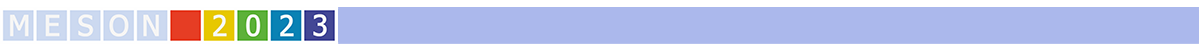#MESON2023

Jun 22 – 27, 2023
Auditorium Maximum
Europe/Warsaw timezone

## A Dalitz-Plot Analysis of the $\omega\to 3\pi$ Decay

Jun 23, 2023, 6:10 PM
20m
Medium lecture hall (B) (Auditorium Maximum)

Parallel

### Speaker

Volker Crede (Florida State University)

### Description

The GlueX Experiment in Hall D at Jefferson Laboratory has accumulated high-statistics samples of photoproduction data off the proton in recent years. Since the light vector mesons $\rho$, $\omega$ and $\phi$ have the same $J^{PC}$ quantum numbers as the incident photon, these mesons are copiously produced in photoproduction and therefore, make GlueX an ideal place to study their production and decay properties. Such studies provide valuable insight into their structure and internal dynamics. Several prominent meson resonances are known to have a dominant three-pion decay mode. At low energies, the light meson decays $\eta\to 3\pi$ and $\phi\to 3\pi$ have been extensively studied and experimental results of their Dalitz-plot distributions are satisfactorily explained by theoretical approaches. In particular, within the dispersive models, the description of the Dalitz plot for vector-to-pseudoscalar decays has shown good agreement with experimental results for the $\phi\to 3\pi$ decay but cannot accurately describe the data for $\omega\to 3\pi$. For the latter, given that the nominal $\rho\pi$ threshold is above the mass of the $\omega$, the distribution of events in the Dalitz plot is rather smooth and, therefore, it can be efficiently parametrized in the experimental analyses by a low-order polynomial in the Dalitz-plot variables. The coefficient $\alpha$ of the leading term in the Dalitz-plot polynomial expansion is consistent with the dominance of the $\rho$-meson contribution, even though it lies outside the kinematical boundary. In this talk, I will present preliminary results of a high-statistics $\omega\to 3\pi$ Dalitz-plot analysis from GlueX. The available statistics exceeds the number of events in a recent BESIII analysis by more than an order of magnitude and allows us to also substantially reduce the uncertainty of the next-to-leading order Dalitz-plot parameters.

Collaboration The GlueX Collaboration

### Primary author

Volker Crede (Florida State University)